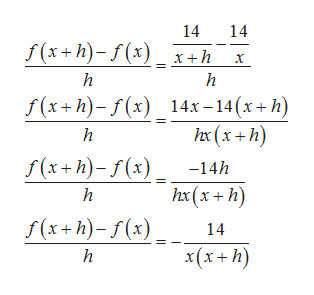# f(x+h)-f(x)Simplify the difference quotientfor the given functionh14f(x)х

Question
3 views
check_circle

Step 1

Given function is

Step 2

Firstly, we find f(x+h) by replacing x by x+h in f(x)

Step 3

By substituting f(x) and f(x+h), a...help_outlineImage Transcriptionclose14 14 S(x+h)-/(х) _хth х h h f(x+h)-f(*) _14х - 14(х+h) hx(x+h) h Г(х+h)-r(%) -14h hx (х + h) h Г(x+п)-/(х) 14 x(х+ h) h fullscreen

### Want to see the full answer?

See Solution

#### Want to see this answer and more?

Solutions are written by subject experts who are available 24/7. Questions are typically answered within 1 hour.*

See Solution
*Response times may vary by subject and question.
Tagged in

### Functions